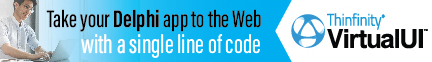DelphiBasicsNegCurrFormatVariable Defines negative amount formatting in currency displays SysUtils unit
 var NegCurrFormat : Byte;
Description
The NegCurrFormat variable defines how negative currency amounts are formatted by such functions as FloatToStr and CurrToStr.

It is in effect an enumerated type, but with no names for the values. It is easiest to show their meanings by example, given below with an amount 1.23 and ? as the CurrencyString:

 ?0 = (?1.23) ?1 = -?1.23 ?2 = ?-1.23 ?3 = ?1.23- ?4 = (1.23?) ?5 = -1.23? ?6 = 1.23-? ?7 = 1.23?- ?8 = -1.23 ? ?9 = -? 1.23 10 = 1.23 ?- 11 = ? 1.23- 12 = ? -1.23 13 = 1.23- ? 14 = (? 1.23) 15 = (1.23 ?)
Notes
NegCurrFormat = LOCALE_INEGCURR by default.
Related commands
 CurrencyDecimals Defines decimal digit count in the Format function CurrencyFormat Defines currency string placement in curr display functions CurrToStrF Convert a currency value to a string with formatting DecimalSeparator The character used to display the decimal point Format Rich formatting of numbers and text into a string ThousandSeparator The character used to display the thousands separatorDownload this web site as a Windows program.